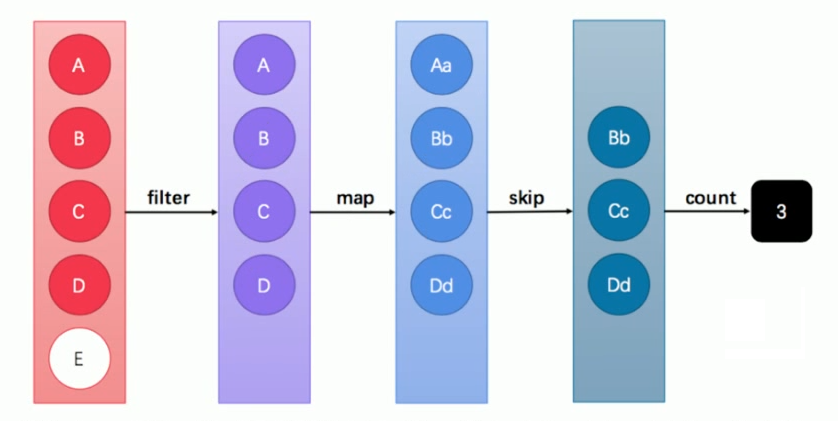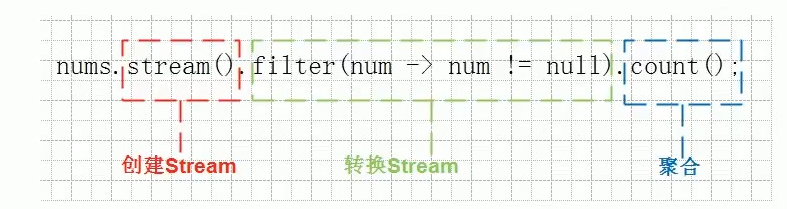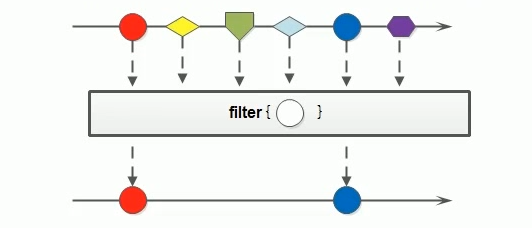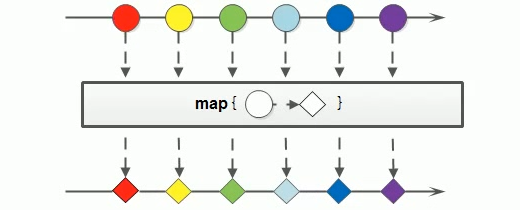Java学习笔记（十一）：Stream流

## 1 引言

### 1.1 传统集合的多步遍历代码

import java.util.ArrayList;
import java.util.List;
public class Demo01ForEach {
public static void main(String[] args) {
List<String> list = new ArrayList<>();
for (String name : list) {
System.out.println(name);
}
}
}

### 1.2 循环遍历的弊端

Java 8的Lambda让我们可以更加专注于做什么（What），而不是怎么做（How），这点此前已经结合内部类进行了对比说明。现在，我们仔细体会一下上例代码，可以发现：

• for循环的语法就是“怎么做
• for循环的循环体才是“做什么

1. 将集合A根据条件一过滤为子集B
2. 然后再根据条件二过滤为子集C

import java.util.ArrayList;
import java.util.List;
public class Demo02NormalFilter {
public static void main(String[] args) {
List<String> list = new ArrayList<>();
List<String> zhangList = new ArrayList<>();
for (String name : list) {
if (name.startsWith("张")) {
}
}
List<String> shortList = new ArrayList<>();
for (String name : zhangList) {
if (name.length() == 3) {
}
}
for (String name : shortList) {
System.out.println(name);
}
}
}

1. 首先筛选所有姓张的人；
2. 然后筛选名字有三个字的人；
3. 最后进行对结果进行打印输出。

### 1.3 Stream的更优写法

import java.util.ArrayList;
import java.util.List;
public class Demo03StreamFilter {
public static void main(String[] args) {
List<String> list = new ArrayList<>();

list.stream()
.filter(s ‐> s.startsWith("张"))
.filter(s ‐> s.length() == 3)
.forEach(System.out::println);
}
}

## 2 流式思想概述Stream（流）是一个来自数据源的元素队列

• 元素是特定类型的对象，形成一个队列。 Java中的Stream并不会存储元素，而是按需计算。
• 数据源 流的来源。 可以是集合，数组 等。

• Pipelining: 中间操作都会返回流对象本身。 这样多个操作可以串联成一个管道， 如同流式风格（fluent style）。 这样做可以对操作进行优化， 比如延迟执行(laziness)和短路( short-circuiting)。
• 内部迭代： 以前对集合遍历都是通过Iterator或者增强for的方式, 显式的在集合外部进行迭代， 这叫做外部迭代。 Stream提供了内部迭代的方式，流可以直接调用遍历方法。

## 3 获取流

java.util.stream.Stream<T>是Java 8新加入的最常用的流接口（这并不是一个函数式接口）。获取一个流非常简单，有以下几种常用的方式：

• 所有的Collection集合都可以通过 stream默认方法获取流；

• Stream接口的静态方法of 可以获取数组对应的流。

### 3.1 根据Collection获取流

import java.util.*;
import java.util.stream.Stream;
public class Demo04GetStream {
public static void main(String[] args) {

List<String> list = new ArrayList<>();
Stream<String> stream1 = list.stream();

Set<String> set = new HashSet<>();
Stream<String> stream2 = set.stream();

Vector<String> vector = new Vector<>();
Stream<String> stream3 = vector.stream();
}
} 

### 3.2 根据Map获取流

java.util.Map 接口不是 Collection 的子接口，且其K-V数据结构不符合流元素的单一特征，所以获取对应的流需要分key、value或entry等情况：

import java.util.HashMap;
import java.util.Map;
import java.util.stream.Stream;
public class Demo05GetStream {
public static void main(String[] args) {

Map<String, String> map = new HashMap<>();
Stream<String> keyStream = map.keySet().stream();
Stream<String> valueStream = map.values().stream();
Stream<Map.Entry<String, String>> entryStream = map.entrySet().stream();
}
}

### 3.3 根据数组获取流

import java.util.stream.Stream;
public class Demo06GetStream {
public static void main(String[] args) {

String[] array = { "张无忌", "张翠山", "张三丰", "张一元" };
Stream<String> stream = Stream.of(array);

Stream<Integer> stream1 = Stream.of(1,2,3,4,5);
}
}  

## 4 常用方法• 延迟方法：返回值类型仍然是 Stream 接口自身类型的方法，因此支持链式调用。（除了终结方法外，其余方法均为延迟方法。）
• 终结方法：返回值类型不再是 Stream 接口自身类型的方法，因此不再支持类似 StringBuilder 那样的链式调用。本小节中，终结方法包括 count 和 forEach 方法。

### 4.1 逐一处理：forEach

void forEach(Consumer<? super T> action);


#### 复习Consumer接口

java.util.function.Consumer<T>接口是一个消费型接口。
Consumer接口中包含抽象方法void accept(T t)，意为消费一个指定泛型的数据。

#### 基本使用

forEach方法用来遍历流中的数据，是一个终结方法，遍历之后就不能再调用流的其他方法。

import java.util.stream.Stream;
public class Demo12StreamForEach {
public static void main(String[] args) {
Stream<String> stream = Stream.of("张无忌", "张三丰", "周芷若");
stream.forEach(name‐> System.out.println(name));
}
}

### 4.2 过滤：filter

Stream<T> filter(Predicate<? super T> predicate);


#### 复习Predicate接口

boolean test(T t);#### 基本使用

Stream流中的 filter 方法基本使用的代码如

import java.util.stream.Stream;
public class Demo07StreamFilter {
public static void main(String[] args) {
Stream<String> original = Stream.of("张无忌", "张三丰", "周芷若");
Stream<String> result = original.filter(s ‐> s.startsWith("张"));
}
}

Stream流属于管道流，只能被使用一次，使用完便转到下一个流，上一个流就会关闭。

### 4.3 映射：map

<R> Stream<R> map(Function<? super T, ? extends R> mapper);#### 复习Function接口

R apply(T t);


#### 基本使用

Stream流中的 map 方法基本使用的代码如：

import java.util.stream.Stream;
public class Demo08StreamMap {
public static void main(String[] args) {
Stream<String> original = Stream.of("10", "12", "18");
Stream<Integer> result = original.map(str‐>Integer.parseInt(str));
}
}

### 4.4 统计个数：count

long count();


import java.util.stream.Stream;
public class Demo09StreamCount {
public static void main(String[] args) {
Stream<String> original = Stream.of("张无忌", "张三丰", "周芷若");
Stream<String> result = original.filter(s ‐> s.startsWith("张"));
System.out.println(result.count()); // 2
}
}

#### 取用前几个：limit

limit 方法可以对流进行截取，只取用前n个。方法签名：

Stream<T> limit(long maxSize);


import java.util.stream.Stream;
public class Demo10StreamLimit {
public static void main(String[] args) {
Stream<String> original = Stream.of("张无忌", "张三丰", "周芷若");
Stream<String> result = original.limit(2);
System.out.println(result.count()); // 2
}
}

#### 跳过前几个：skip

Stream<T> skip(long n);


import java.util.stream.Stream;
public class Demo11StreamSkip {
public static void main(String[] args) {
Stream<String> original = Stream.of("张无忌", "张三丰", "周芷若");
Stream<String> result = original.skip(2);
System.out.println(result.count()); // 1
}
}

### 4.5 组合：concat

static <T> Stream<T> concat(Stream<? extends T> a, Stream<? extends T> b)


import java.util.stream.Stream;
public class Demo12StreamConcat {
public static void main(String[] args) {
Stream<String> streamA = Stream.of("张无忌");
Stream<String> streamB = Stream.of("张翠山");
Stream<String> result = Stream.concat(streamA, streamB);
}
}

## 5 练习：集合元素处理（传统方式）

### 题目

1. 第一个队伍只要名字为3个字的成员姓名；存储到一个新集合中。
2. 第一个队伍筛选之后只要前3个人；存储到一个新集合中。
3. 第二个队伍只要姓张的成员姓名；存储到一个新集合中。
4. 第二个队伍筛选之后不要前2个人；存储到一个新集合中。
5. 将两个队伍合并为一个队伍；存储到一个新集合中。
6. 根据姓名创建 Person 对象；存储到一个新集合中。
7. 打印整个队伍的Person对象信息

import java.util.ArrayList;
import java.util.List;
public class DemoArrayListNames {
public static void main(String[] args) {
//第一支队伍
ArrayList<String> one = new ArrayList<>();
//第二支队伍
ArrayList<String> two = new ArrayList<>();
// ....
}
}

public class Person {
private String name;
public Person() {}
public Person(String name) {
this.name = name;
}
@Override
public String toString() {
return "Person{name='" + name + "'}";
}
public String getName() {
return name;
}
public void setName(String name) {
this.name = name;
}
}

### 解答

public class DemoArrayListNames {
public static void main(String[] args) {
List<String> one = new ArrayList<>();
// ...
List<String> two = new ArrayList<>();
// ...
// 第一个队伍只要名字为3个字的成员姓名；
List<String> oneA = new ArrayList<>();
for (String name : one) {
if (name.length() == 3) {
}
}
// 第一个队伍筛选之后只要前3个人；
List<String> oneB = new ArrayList<>();
for (int i = 0; i < 3; i++) {
}
// 第二个队伍只要姓张的成员姓名；
List<String> twoA = new ArrayList<>();
for (String name : two) {
if (name.startsWith("张")) {
}
}
// 第二个队伍筛选之后不要前2个人；
List<String> twoB = new ArrayList<>();
for (int i = 2; i < twoA.size(); i++) {
}
// 将两个队伍合并为一个队伍；
List<String> totalNames = new ArrayList<>();
// 根据姓名创建Person对象；
List<Person> totalPersonList = new ArrayList<>();
for (String name : totalNames) {
}
// 打印整个队伍的Person对象信息。
for (Person person : totalPersonList) {
System.out.println(person);
}
}
}

Person{name='宋远桥'}
Person{name='苏星河'}
Person{name='石破天'}
Person{name='张天爱'}
Person{name='张二狗'}

## 6 练习：集合元素处理（Stream方式）

### 解答

import java.util.ArrayList;
import java.util.List;
import java.util.stream.Stream;
public class DemoStreamNames {
public static void main(String[] args) {
List<String> one = new ArrayList<>();
// ...
List<String> two = new ArrayList<>();
// ...
// 第一个队伍只要名字为3个字的成员姓名；
// 第一个队伍筛选之后只要前3个人；
Stream<String> streamOne = one.stream().filter(s ‐> s.length() == 3).limit(3);
// 第二个队伍只要姓张的成员姓名；
// 第二个队伍筛选之后不要前2个人；
Stream<String> streamTwo = two.stream().filter(s ‐> s.startsWith("张")).skip(2);
// 将两个队伍合并为一个队伍；
// 根据姓名创建Person对象；
// 打印整个队伍的Person对象信息。
Stream.concat(streamOne, streamTwo).map(Person::new).forEach(System.out::println);
//Stream.concat(streamOne,streamTwo).map(name->new Person(name)).forEach(p->System.out.println(p))
}
}

Person{name='宋远桥'}
Person{name='苏星河'}
Person{name='石破天'}
Person{name='张天爱'}
Person{name='张二狗'}

目录